# How to Find the Longest Common Prefix in JavaScriptself.__wrap_n=self.__wrap_n||(self.CSS&&CSS.supports("text-wrap","balance")?1:2);self.__wrap_b=(e,r,t)=>{t=t||document.querySelector(`[data-br="\${e}"]`);let n=t.parentElement,a=s=>t.style.maxWidth=s+"px";t.style.maxWidth="";let o,i=n.clientWidth,l=n.clientHeight,c=i/2-.25,f=i+.5;if(i){for(a(c),c=Math.max(t.scrollWidth,c);c+1<f;)o=Math.round((c+f)/2),a(o),n.clientHeight===l?f=o:c=o;a(f*r+i*(1-r))}t.__wrap_o||typeof ResizeObserver<"u"&&(t.__wrap_o=new ResizeObserver(()=>{self.__wrap_b(0,+t.dataset.brr,t)})).observe(n)};self.__wrap_n!=1&&self.__wrap_b(":Rj0pm:",1)

November 8, 2023

The longest common prefix of an array of strings is the initial segment that is common to all strings in the array. In JavaScript, this can be computed through string comparison and iteration techniques.

## Understanding the problem

To find the longest common prefix, we compare the characters of each string at the same index until a mismatch is found. This operation is performed iteratively or recursively, stopping when the shortest string's length is reached or a differing character is encountered.

## Implementing a solution

Here's an approach to solving this problem using JavaScript:

```function findLongestCommonPrefix(strs) {
if (!strs.length) return '';

let prefix = strs;

for (let i = 1; i < strs.length; i++) {
while (strs[i].indexOf(prefix) !== 0) {
prefix = prefix.substring(0, prefix.length - 1);
if (!prefix) return '';
}
}

return prefix;
}```

## How the solution works

1. Initialize the prefix to the first string in the array.
2. Iterate over the array of strings.
3. Use the `indexOf` function to check if the current string contains the prefix at the start.
4. If not, reduce the prefix by one character from the end and repeat the process.
5. If the prefix is empty or the prefix is found at the start of each string, return the prefix.

## Horizontal scanning

The horizontal scanning technique involves taking the first string as the initial prefix and then comparing it with the next string, shortening the prefix from the end with each mismatch. This process is continued for all strings in the array.

## Time complexity

The time complexity of this solution is (O(S)), where (S) is the sum of all characters in all strings. The worst-case scenario occurs when all strings are identical.

## Space complexity

The space complexity is (O(1)), as the space used by the algorithm is constant and does not grow with the size of the input array.

## Test cases

Let's validate our function with a few test cases:

```console.log(findLongestCommonPrefix(["flower","flow","flight"])); // "fl"
console.log(findLongestCommonPrefix(["dog","racecar","car"])); // ""
console.log(findLongestCommonPrefix(["interspecies","interstellar","interstate"])); // "inters"```

## Tips for optimization

• Early stopping if a string is empty or if the prefix is reduced to zero length at any point.
• Using a divide and conquer technique can improve performance on large datasets.
• Implementing a trie data structure might yield better performance for a large number of strings with a complex set of common prefixes.

## How to find the longest common prefix string amongst an array of strings in JavaScript

To find the longest common prefix among an array of strings in JavaScript, you can use the vertical scanning method. This technique compares characters from the top down at the same index of each string.

```function longestCommonPrefix(strs) {
if (strs.length === 0) return '';

for (let i = 0; i < strs.length; i++) {
const char = strs[i];
for (let j = 1; j < strs.length; j++) {
if (i === strs[j].length || strs[j][i] !== char) {
return strs.substring(0, i);
}
}
}
return strs;
}```

### Vertical scanning explained

1. Check if the array is empty; if so, return an empty string.
2. Loop through each character of the first string.
3. Compare the current character with the corresponding character of all other strings.
4. If a mismatch is found or the end of a string is reached, return the common prefix up to the previous character.
5. If the loop completes without a mismatch, the entire first string is the longest common prefix.

### Test cases for vertical scanning

You can verify the correctness of the vertical scanning function with these test cases:

```console.log(longestCommonPrefix(["alpine", "altitude", "algorithm"])); // "al"
console.log(longestCommonPrefix(["blueberry", "blue", "bluetooth"])); // "blu"
console.log(longestCommonPrefix(["zebra", "dog", "duck"])); // ""
console.log(longestCommonPrefix(["prefix", "preform", "premise"])); // "pre"```

### When to use vertical scanning

Vertical scanning is particularly effective when:

• The array has a very long string and the prefix is short because it stops checking once the shortest string's length is reached.
• The number of strings is significantly greater than the length of the shortest string.

# Ship faster, worry less with Basedash

You're busy enough with product work to be weighed down building, maintaining, scoping and developing internal apps and admin panels. Forget all of that, and give your team the admin panel that you don't have to build. Launch in less time than it takes to run a standup.

You're busy enough with product work to be weighed down building, maintaining, scoping and developing internal apps and admin panels. Forget all of that, and give your team the admin panel that you don't have to build. Launch in less time than it takes to run a standup.

You're busy enough with product work to be weighed down building, maintaining, scoping and developing internal apps and admin panels. Forget all of that, and give your team the admin panel that you don't have to build. Launch in less time than it takes to run a standup.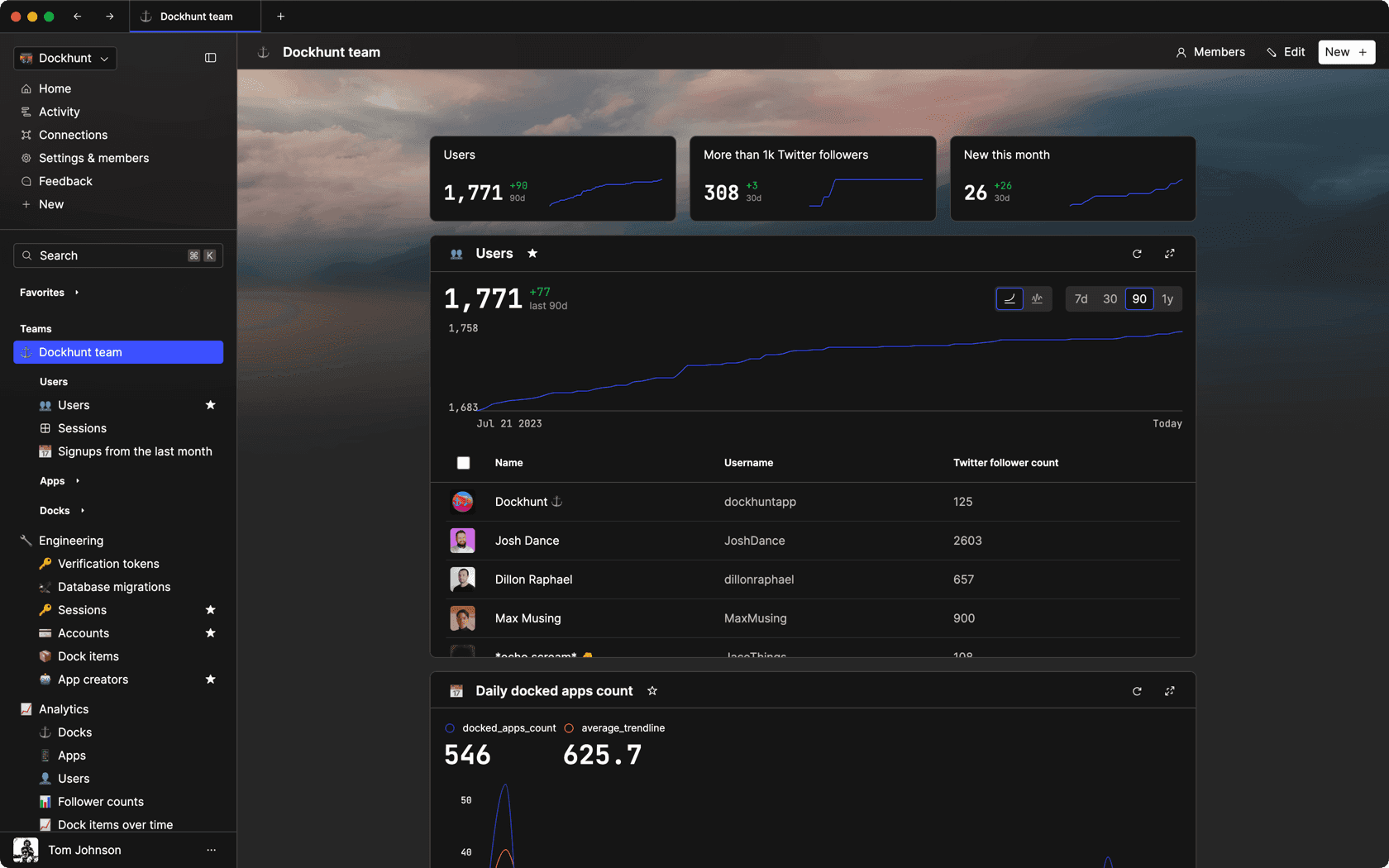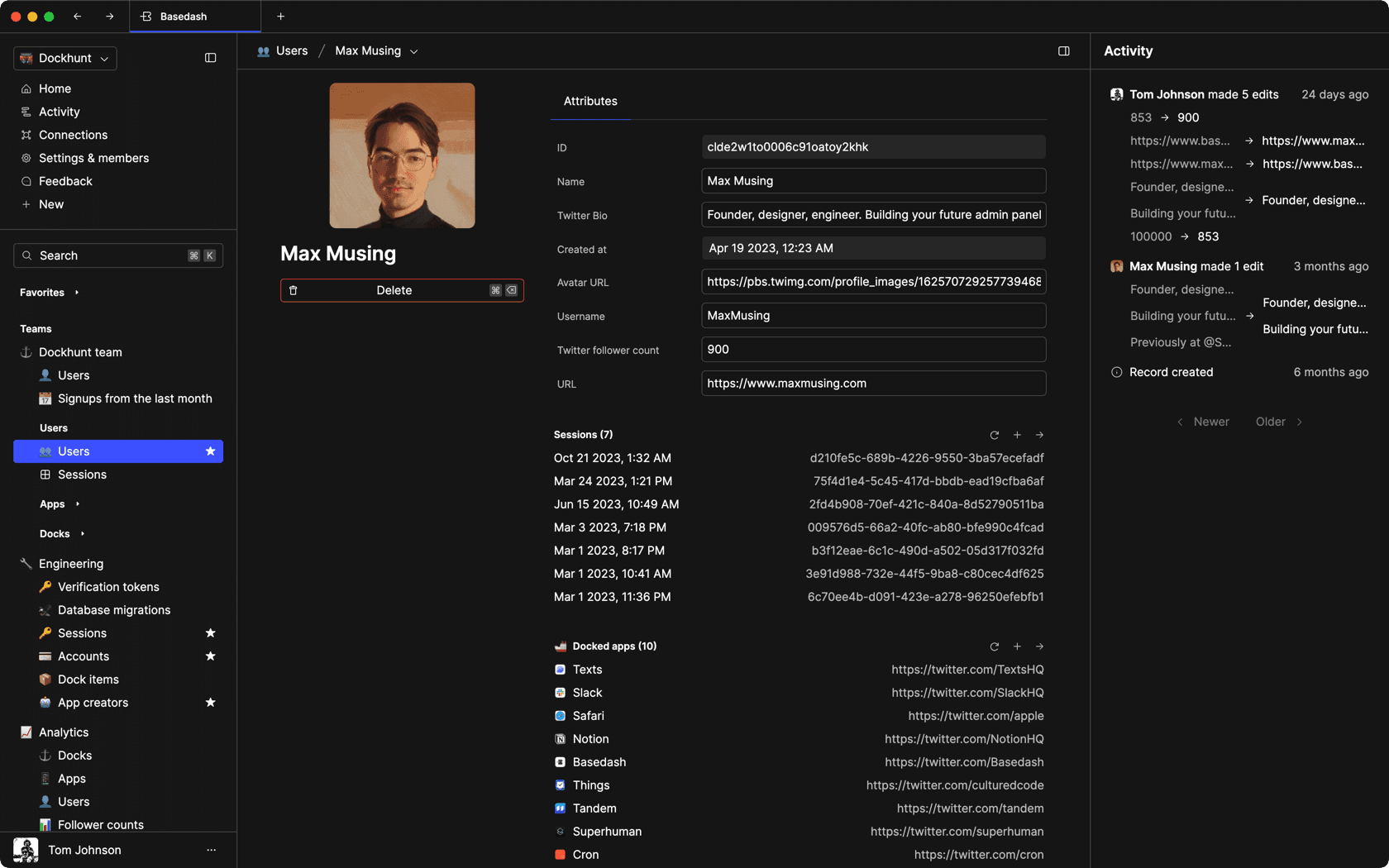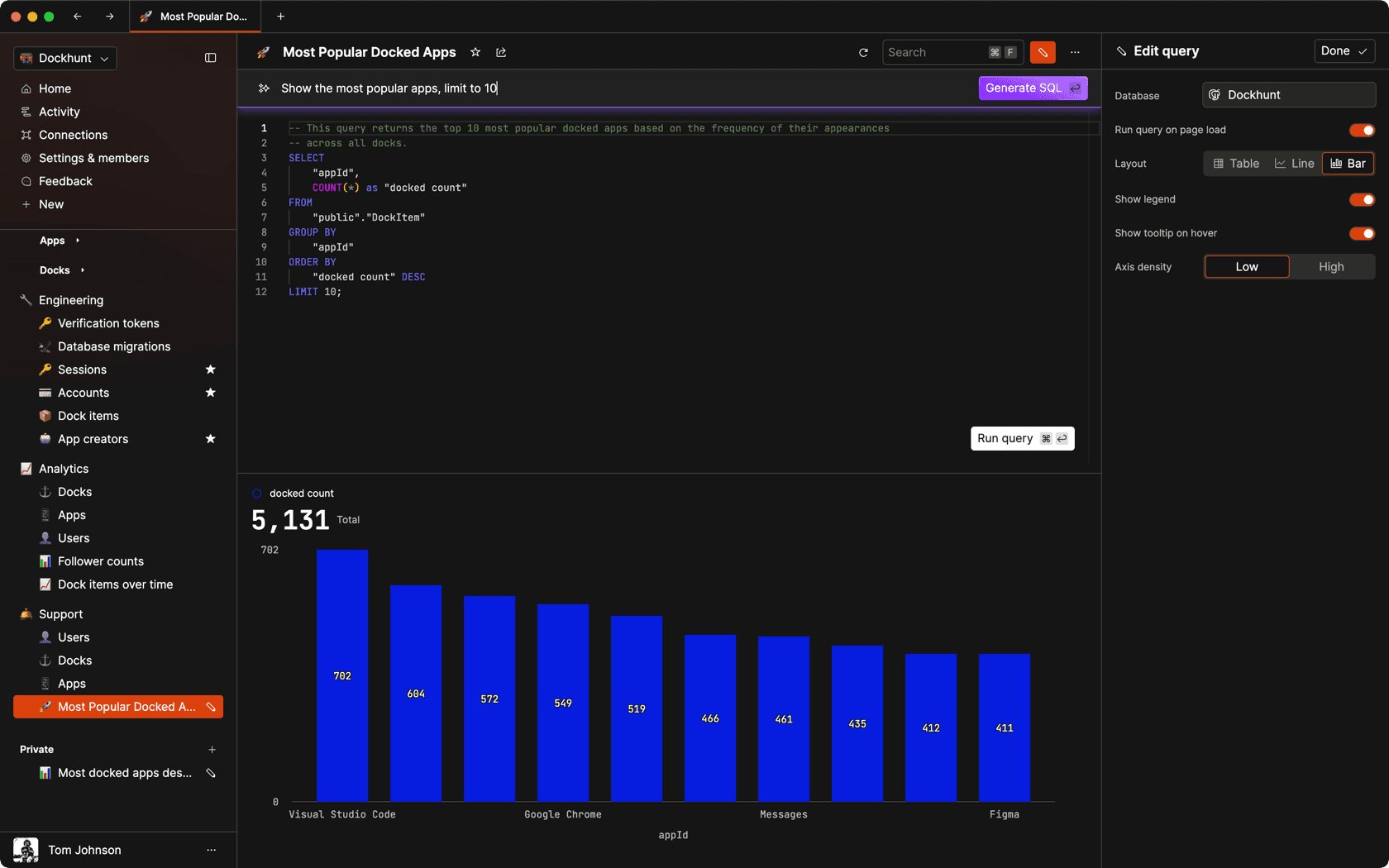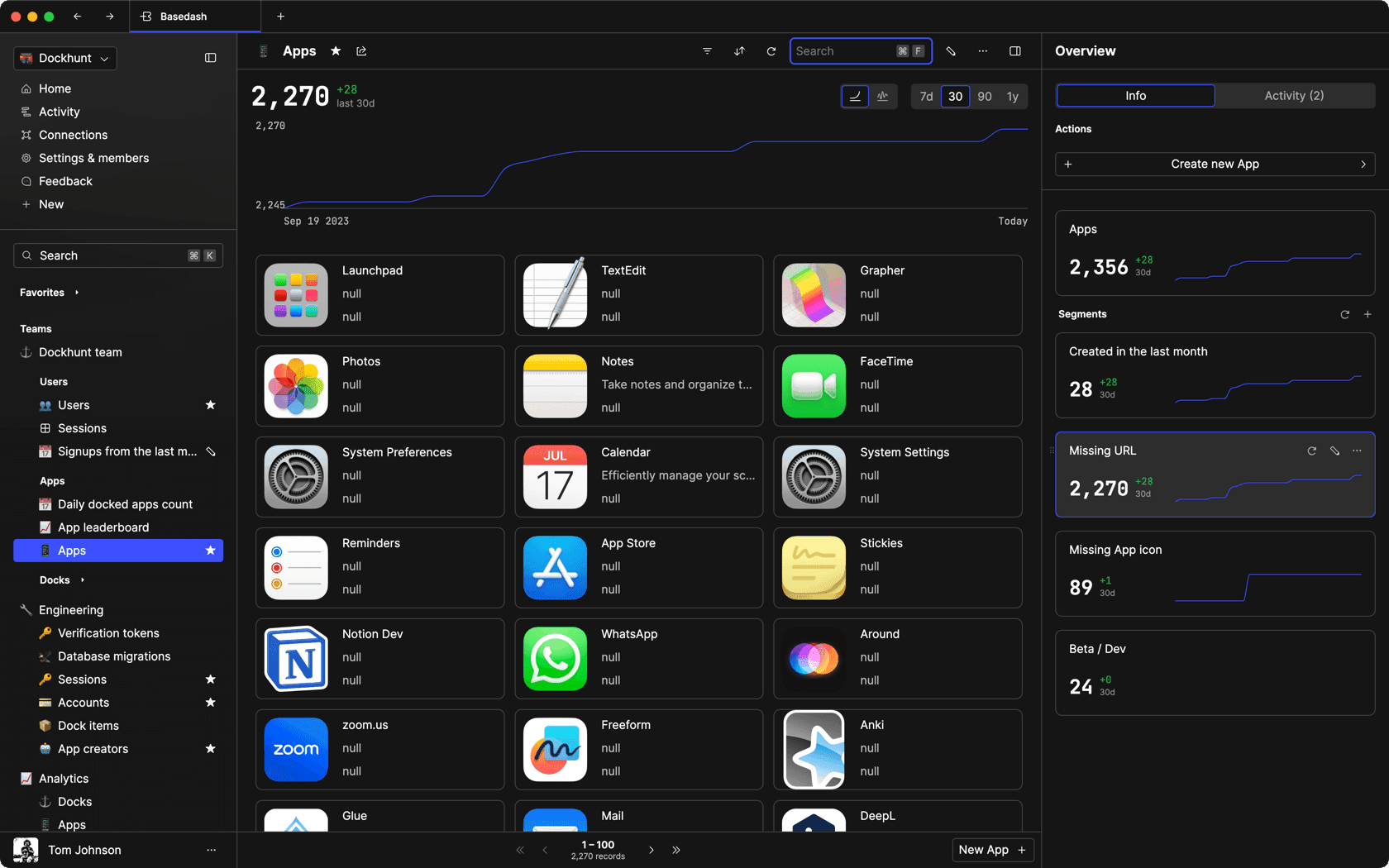### Dashboards and charts

Effortlessly make charts and create a space for your team to work together towards shared goals and metrics.

### SQL composer with AI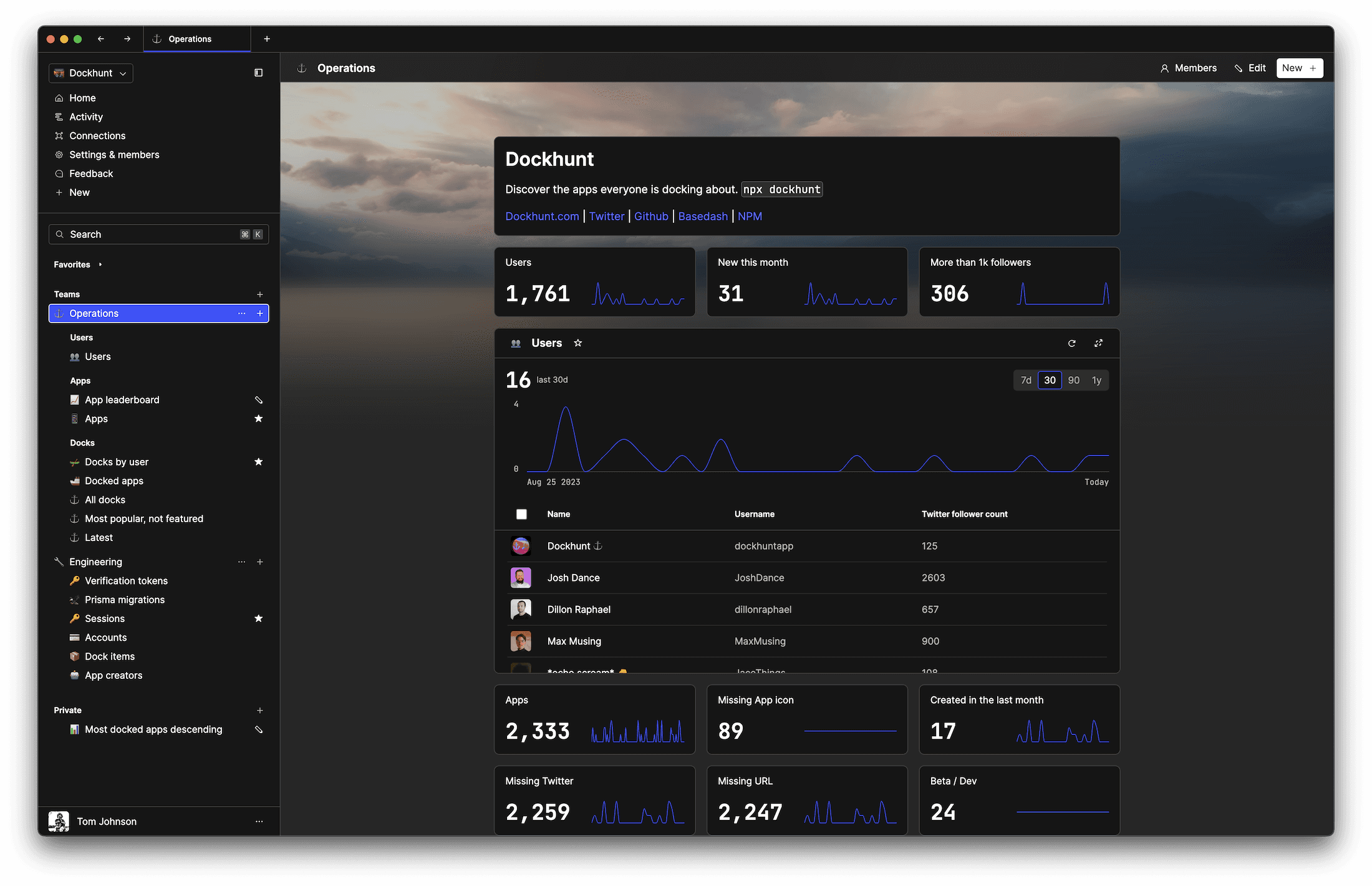SOC II
Type 2 Certified

### Features

Proudly carbon-neutral.SOC II
Type 2 Certified

### Features

Proudly carbon-neutral.SOC II
Type 2 Certified